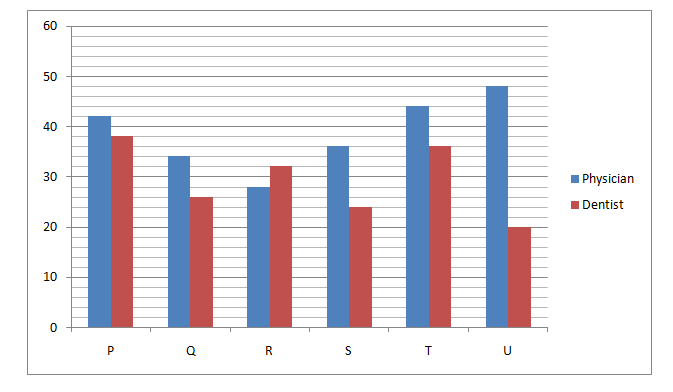# SBI PO Prelims Quantitative Aptitude Questions 2019 (Day-34)

Dear Aspirants, Our IBPS Guide team is providing new series of Quantitative Aptitude Questions for SBI PO 2019 so the aspirants can practice it on a daily basis. These questions are framed by our skilled experts after understanding your needs thoroughly. Aspirants can practice these new series questions daily to familiarize with the exact exam pattern and make your preparation effective.

[WpProQuiz 6130]

### Click Here for SBI PO Pre 2019 High-Quality Mocks Exactly on SBI Standard

Directions (Q. 1 – 5): What value should come in place of (?) in the following questions?

1) 13824 + (10648) % of 150 = ? – 113

a) 170

b) 155

c) 140

d) 125

e) None of these

2) 42 % of 1800 + (9/7) of 1421 – 189 ÷ 9 × 3 = ?

a) 2140

b) 1860

c) 2520

d) 1580

e) None of these

3) 15 × 3 × (45 – 33) + 176 ÷ 4 ÷ 2 – 122 = ?

a) 532

b) 284

c) 376

d) 418

e) None of these

4) 1040 ÷ [(1/8) of (23 + 17) + 15] = ?

a) 38

b) 52

c) 74

d) 66

e) None of these

5) 7 ¾ + 3 ½ + 4 ¼ – 3 5/8 = ? + 2 3/8

a) 8 5/8

b) 10 3/8

c) 9 ½

d) 10 ¾

e) None of these

Directions (Q. 6 – 10) DI

Directions (Q. 6 – 10): Study the following information carefully and answer the given questions.

The following line graph shows the total number of doctors (Dentists + Physicians) in 6 different cities. (In hundreds)6) Find the difference between the total number of physician in city P and R together to that of total number of dentist in city Q and S together?

a) 2000

b) 3400

c) 2200

d) 3800

e) None of these

7) Find the ratio between the total number of doctors in city R and T together to that of total number of doctors in city S and U together?

a) 28 : 17

b) 13 : 5

c) 42 : 23

d) 35 : 32

e) None of these

8) Total number of physicians in city Q and S together is what percentage more than the total number of dentists in the same cities?

a) 25 %

b) 40 %

c) 50 %

d) 15 %

e) None of these

9) Find the average number of dentists in all the given cities except the city T?

a) 2400

b) 3000

c) 2800

d) 3200

e) None of these

10) Total number of doctors in city P is approximately what percentage of total number of doctors in city R?

a) 135 %

b) 120 %

c) 95 %

d) 150 %

e) 80 %

24 + 22 % of 150 = x – 113

24 + (22/100)*150 + 113 = x

x = 24 + 33 + 113 = 170

(42/100) * 1800 + (9/7) * 1421 – (189/9) * 3 = x

756 + 1827 – 63 = x

x = 2520

15 × 3 × (45 – 33) + 176 ÷ 4 ÷ 2 – 122 = x

15 × 3 × 12 + 22 – 144 = x

540 + 22 – 144 = x

x = 418

1040 ÷ [(1/8)*40 + 15] = x

X = 1040 / (5 + 15)

X = 1040/20 = 52

7 ¾ + 3 ½ + 4 ¼ – 3 5/8 – 2 3/8 = x

X = (7 + 3 + 4 – 3 – 2) (3/4 + ½ + ¼ – 5/8 – 3/8)

X = 9 (1/2)

The total number of physician in city P and R together

= > 4200 + 2800 = 7000

The total number of dentist in city Q and S together

= > 2600 + 2400 = 5000

Required difference = 7000 – 5000 = 2000

The total number of doctors in city R and T together

= > 2800 + 3200 + 4400 + 3600 = 14000

The total number of doctors in city S and U together

= > 3600 + 2400 + 4800 + 2000 = 12800

Required ratio = 14000 : 12800 = 35 : 32

Total number of physicians in city Q and S together

= > 3400 + 3600 = 7000

Total number of dentists in city Q and S together

= > 2600 + 2400 = 5000

Required % = [(7000 – 5000)/5000]*100 = 40 %

Required average

= > (3800 + 2600 + 3200 + 2400 + 2000)/5

= > 14000/5 = 2800